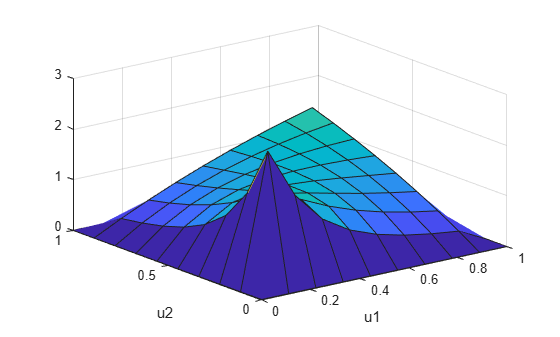# copulapdf

Copula probability density function

## Syntax

``y = copulapdf('Gaussian',u,rho)``
``y = copulapdf('t',u,rho,nu)``
``y = copulapdf(family,u,alpha)``

## Description

````y = copulapdf('Gaussian',u,rho)` returns the probability density of the Gaussian copula with linear correlation parameters, `rho`, evaluated at the points in `u`.```
````y = copulapdf('t',u,rho,nu)` returns the probability density of the t copula with linear correlation parameters, `rho`, and degrees of freedom parameter, `nu`, evaluated at the points in `u`.```

example

````y = copulapdf(family,u,alpha)` returns the probability density of the bivariate Archimedean copula of the type specified by `family`, with scalar parameter, `alpha`, evaluated at the points in `u`.```

## Examples

collapse all

Define two 10-by-10 matrices containing the values at which to compute the pdf.

```u = linspace(0,1,10); [u1,u2] = meshgrid(u,u);```

Compute the pdf of a Clayton copula that has an alpha parameter equal to 1, at the values in `u`.

`y = copulapdf('Clayton',[u1(:),u2(:)],1);`

Plot the pdf as a surface, and label the axes.

```surf(u1,u2,reshape(y,10,10)) xlabel('u1') ylabel('u2')```## Input Arguments

collapse all

Values at which to evaluate the pdf, specified as a matrix of scalar values in the range [0,1]. If `u` is an n-by-p matrix, then its values represent n points in the p-dimensional unit hypercube. If `u` is an n-by-2 matrix, then its values represent n points in the unit square.

If you specify a bivariate Archimedean copula type (`'Clayton'`, `'Frank'`, or `'Gumbel'`), then `u` must be an n-by-2 matrix.

Data Types: `single` | `double`

Linear correlation parameters for the copula, specified as a scalar value or matrix of scalar values.

• If `u` is an n-by-p matrix, then `rho` is a p-by-p correlation matrix.

• If `u` is an n-by-2 matrix, then `rho` can be a scalar correlation coefficient.

Data Types: `single` | `double`

Degrees of freedom for the t copula, specified as a positive integer value.

Data Types: `single` | `double`

Bivariate Archimedean copula family, specified as one of the following.

 `'Clayton'` Clayton copula `'Frank'` Frank copula `'Gumbel'` Gumbel copula

Bivariate Archimedean copula parameter, specified as a scalar value. Permitted values for `alpha` depend on the specified copula family.

Copula FamilyPermitted Alpha Values
`'Clayton'`[0,∞)
`'Frank'`(-∞,∞)
`'Gumbel'`[1,∞)

Data Types: `single` | `double`

## Output Arguments

collapse all

Probability density function, evaluated at the values in `u`, returned as a vector of scalar values.

## Version History

Introduced in R2006a